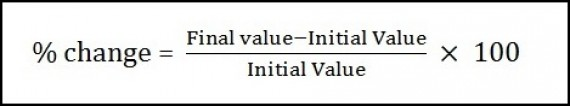# Dealing with Percentages – Part 1A percentage, for the lack of a better definition, is a number or ratio as a fraction of 100. Probably its oldest application, like so many other things in today’s world, was at the time of the Roman Empire. Augustus, founder of the Roman Empire and its first Emperor, levied a tax of 1/100 on goods sold at auction known as centesima rerum venalium. Computation with these fractions was similar to computing percentages. The word itself is derived from the Latin per centum meaning “by the hundred”. The percent sign evolved by gradual contraction of the phrase “per cento”. The “per” was often abbreviated as “p.” and eventually disappeared entirely. The “cento” was contracted to two circles separated by a horizontal line from which the modern “%” is derived. But enough about history…let us come back to the present and some practical uses of percentages.

Percentages as Fractions:

When it comes to calculating percentages, one of the best tips is to make the use of fractions. Given below is a table which can help you get started. There is no better way to remember these values than to start using them in your daily calculations.

 Fraction Percentage Fraction Percentage 1/2 50% 1/9 11.11% 1/3 33.33% 1/10 10% 1/4 25% 1/11 9.09% 1/5 20% 1/12 8.33% 1/6 16.66% 1/13 7.69% 1/7 14.28% 1/14 7.14% 1/8 12.5% 1/15 6.66%

Example:

Find 28.5% of 476

(a) 135.66      (b) 136           (c) 136.28      (d) 136.43

As you can see here the options are really close and you may think that you would have to calculate the actual value. But if you are well versed with the table given above, you can save some effort and time. The seconds you save here may be vital in the exam.

To calculate 28.5%, I urge you to have a closer look at 1/7.

1/7 represents 14.28%, so 2/7 would represent 28.56% which is freakishly close to the percentage that we are looking for.

Also, calculating 2/7 of 476 is not that difficult. 476 is divisible by 7 – the answer it is 68. So, 2/7 of 476 will be 136.

Now, we know that 2/7 is 28.56% and we also know that that it is 136.

Our answer will be little less than 136 as we are trying to find out 28.5% which is a little less than 28.56%. So, our answer would be 135.66. Option (a)

Percentage Change:

To find the percentage change, you can use the formula:A lot of people make mistakes in calculating values related to the given formula because they do not calculate % change based upon the initial value.

Example:

My current salary is Rs. 1000 a day, which is 25% higher than what it was last year. What was my salary last year?

If you think that the answer to the above question is Rs. 750 per day then you are making the same mistake that a lot of students make. The raise that I got (25%) was not based on my current salary but on the initial value, which was my salary in the previous year.

To calculate it correctly, let us assume my last year’s salary to be Rs. ‘x’ per day.

• A 25% increase means an increase of ¼,
• My current salary is 5/4 of my previous year’s salary.
• This means that my salary last year was 4/5 of my current salary.

My last year’s salary = (4/5)×100 = Rs. 800

Changing Quantities by Percent:

The simplest way to change a number by a given percent is to simply multiply it by the ‘final’ percentage.

That is, to increase a number by x%, simply multiply it by (100 + x)%  or  (100 + x)/100

and to decrease it by x%, simply multiply it by (100 – x)%  or (100 – x)/100

Example:

A piece of paper is in the shape of a right angled triangle and is cut along a line that is parallel to the hypotenuse, leaving a smaller triangle. There was a 35% reduction in the length of the hypotenuse of the triangle. If the area of the original triangle was 34 square inches before the cut, what is the area (in square inches) of the smaller triangle?

(a)  16.665            (b) 16.565      (c) 15.465      (d) 14.365

If the hypotenuse reduced by 35%, it became 100 – 35 = 65% of the original or in other words it became 0.65 times the original. The other two sides also become 0.65 times the original. Area will become (0.65) ^2 = 0.4225 times the original.

New area = 0.4225*34 = 14.365. Option (d)

I hope that you liked this introductory article on ‘Percentages’. In Part 2, I will cover two important concepts:

a)     Successive Percentage Changes

b)     Compensating a Percentage Change

If you have any other ideas or suggestions with respect to concepts that should be covered in ‘Dealing with Percentages – Part 2’, please feel free to use the comment section.

– Ravi Handa(Ravi Handa, an alumnus of IIT Kharagpur, has been working in the CAT Prep sector for the past 7 years. He currently offers online CAT coaching and preparation for CAT 2015)

Other articles by Ravi Handa can be found here

## Ravi Handa

Founder of http://handakafunda.com